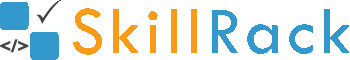#### Paid/Free Events Registration

C PROGRAMMING WEBINARS 50 SESSIONS (TCS/CTS/Wipro Preparation)(PRIME)

(LIVE NOW)

Summary

C PROGRAMMING (TCS/CTS/Wipro Preparation) webinar series provides 50 hours of learning and hands-on programming experience for practice programs. In each webinar session, the related programs will be scheduled for hands-on practice.

SOLUTION is provided for all the webinar programs.

Content: C PROGRAMMING webinar series

C Programming - Hour 001 (C program compilation process - Structure of C program - How to print output - Pretty/formatted printing in C - Variables and Datatypes - Datatype Comparison)
C Programming - Hour 002 (How to accept input - Accepting integer input - Accepting float input - Accepting character and string input - Accepting comma-separated values and other formatted input)
C Programming - Hour 003 (Arithmetic operators - Evaluating expressions - Expression vs Statement - Digit Manipulations)
C Programming - Hour 004 (What is boolean? - Relational operators - Ternary operator)
C Programming - Hour 005 (Unary Operators - If Else Condition - Conditional Printing Problems)

C Programming - Hour 006 (Digit Manipulations - Access digits from the end - Access last X digits - Remove Digits from the end)
C Programming - Hour 007 (Introduction to Conditional Statements - If condition - If Else condition)
C Programming - Hour 008 (Else If Ladder Introduction - Multiple Conditions)
C Programming - Hour 009 (Nested If Else Introduction - Nested Conditions)
C Programming - Hour 010 (Logical Operators - Multiple conditions in if statements)

C Programming - Hour 011 (Switch Statement - Characters and Integers in Switch - Default Case - Matching Range in the switch statement - Do’s and don’ts in the switch statement)
C Programming - Hour 012 (Loop, While Loop Introduction - Automating repetitive tasks)
C Programming - Hour 013 (Traversing digits using while loop - Accessing each digit in an integer)
C Programming - Hour 014 (Digit Manipulations - Conditions on digits)
C Programming - Hour 015 (Advanced Digit Manipulations programs - Number Crunching)

C Programming - Hour 016 (Accepting Unknown Number of Input - Conditionally Scanning Input values - Accepting multiple input values)
C Programming - Hour 017 (For Loop Introduction - Integer range traversals using for loop)
C Programming - Hour 018 (Using conditions in for loop - Logical operators in for loop)
C Programming - Hour 019 (Problems on for loop SET 001)
C Programming - Hour 020 (Problems on for loop SET 002)

C Programming - Hour 021 (Do while loop introduction)
C Programming - Hour 022 (Nested Loops Introduction - Simple grid pattern printing)
C Programming - Hour 023 (Triangular pattern printing structure using nested loops)
C Programming - Hour 024 (Problems on pattern printing)
C Programming - Hour 025 (Infinite Loop - Loop controls using break and continue statements)

C Programming - Hour 026 (Array Introduction, Array Traversal Forward and Reverse)
C Programming - Hour 027 (Array Neighbouring Elements Manipulations)
C Programming - Hour 028 (Circular Traversal - Array Algorithms - Array Rotations)
C Programming - Hour 029 (String Introduction - Char Array - null character)
C Programming - Hour 030 (String Manipulations and String functions)

C Programming - Hour 031 (Accepting String without space as input, Advanced String Input)
C Programming - Hour 032 (Problems on String)
C Programming - Hour 033 (String Hashing and Efficient Comparison Techniques)
C Programming - Hour 034 (2D Array Introduction)
C Programming - Hour 035 (Matrix Traversals - Horizontally, Vertically & Diagonally)

C Programming - Hour 036 (Border Elements - Zigzag Matrix Traversal programs)
C Programming - Hour 037 (Character Matrix - Views of Matrix programs)
C Programming - Hour 038 (Bitwise Operators and their usage)
C Programming - Hour 039 (Function Introduction - Return Value - Arguments)
C Programming - Hour 040 (Arrays and String in Function)

C Programming - Hour 041 (Passing matrix to a function)
C Programming - Hour 042 (Recursion - Simulating Loop using Recursion - Problem solving techniques using Recursion)
C Programming - Hour 043 (Pointers - Double Pointer - Multiple Pointer)
C Programming - Hour 044 (Pointer to Array, Array of Pointers)
C Programming - Hour 045 (Dynamic Memory Allocation - Heap vs Stack Memory)

C Programming - Hour 046 (Pointer to Matrix, Passing matrix as pointer to a function, Traversing matrix with pointer)
C Programming - Hour 047 (File Handling - Opening file in C - Reading integers - Reading multiple values)
C Programming - Hour 048 (File Handling -  Writing to a file - writing multiple values to a file)
C Programming - Hour 049 (Structures - Union)
C Programming - Hour 050 (Structure Pointer - Macros)

FAQ:

1) Can I view the video explanation multiple times?

2) Can I view the video explanation at any time (24/7) from the event start date to the end date?

3) Can I solve the program after the webinar end date/time?

4) Is the solution provided for all the webinar programs?

5) Can I view the video explanation after the webinar end date/time?